# Spatiocyte Modules¶

In Spatiocyte modules, the unit of numeric values is given in meters, seconds, radians and molecule numbers. A Spatiocyte model file created using the E-Cell Model (EM) language is shown in Figure 2. The file contains the wildtype Escherichia coli MinDE cytokinesis regulation model that was reported in (Arjunan and Tomita, 2010). A schematic representation of the model is given in Figure 4. Python script examples to build models with more complex compartments are provided in Figures 5 and 6. Figures 7 and 8 illustrate 3D visualizations of the resulting models.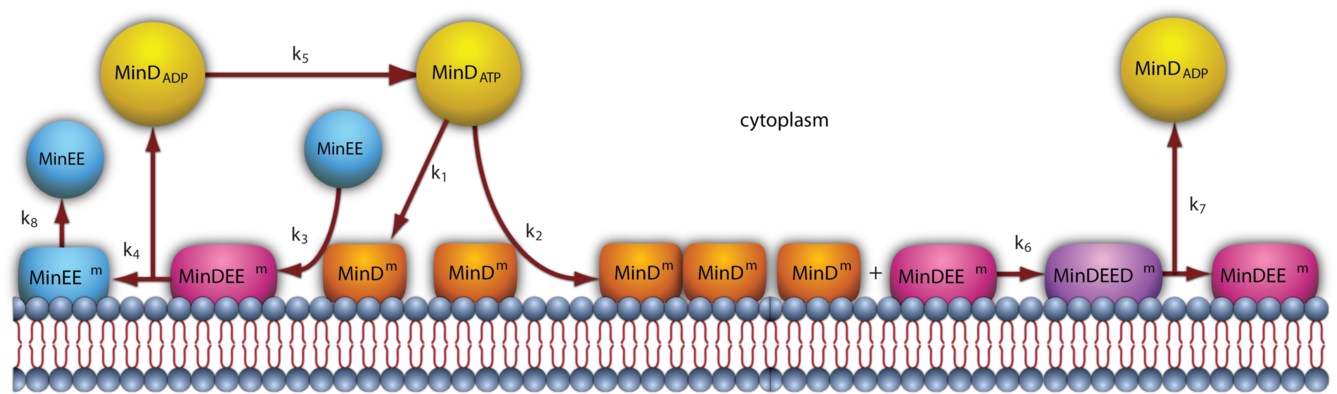Figure 4: A schematic representation of the MinDE model.

## Compartment¶

Compartments are defined hierarchically and follow the format used by the E-Cell System version 3 (see the E-Cell Simulation Environment Version 3 User’s Manual for details). Each sub-compartment within a parent compartment is created according to the alphabetical order of the compartment names. Predefined Variables that specify the Compartment properties include DIMENSION, GEOMETRY, LENGTHX, LENGTHY, LENGTHZ, ORIGINX, ORIGINY, ORIGINZ, ROTATEX, ROTATEY, ROTATEZ, XYPLANE, XZPLANE, YZPLANE, VACANT, DIFFUSIVE and REACTIVE. Examples of these variable definitions can be seen in Figures 2 (lines 4-7 and 33-34), 5 (lines 5-8, 17-24, 31-32, 41-49 and 52-54) and 6 (lines 46-49, 59-60, 63-69 and 71-72).

 ``` 1 2 3 4 5 6 7 8 9 10 11 12 13 14 15 16 17 18 19 20 21 22 23 24 25 26 27 28 29 30 31 32 33 34 35 36 37 38 39 40 41 42 43 44 45 46 47 48 49 50 51 52 53 54 55``` ```# Example of python scripting to create a neuron with 5 minor processes theSimulator.createStepper('SpatiocyteStepper', 'SS').VoxelRadius = 10e-8 # Create the root container compartment using the default Cuboid geometry: theSimulator.rootSystem.StepperID = 'SS' theSimulator.createEntity('Variable', 'Variable:/:LENGTHX').Value = 61e-6 theSimulator.createEntity('Variable', 'Variable:/:LENGTHY').Value = 25e-6 theSimulator.createEntity('Variable', 'Variable:/:LENGTHZ').Value = 5.5e-6 theSimulator.createEntity('Variable', 'Variable:/:VACANT') logger = theSimulator.createEntity('VisualizationLogProcess', 'Process:/:logger') logger.LogInterval = 1 logger.VariableReferenceList = [['\_', 'Variable:/Soma/Membrane:VACANT'], ['\_', 'Variable:/Soma:K']] logger.VariableReferenceList = [['\_', 'Variable:/Dendrite%d/Membrane:VACANT' %i] for i in range(5)] populator = theSimulator.createEntity('MoleculePopulateProcess', 'Process:/:populate') populator.VariableReferenceList = [['\_', 'Variable:/Soma:K']] # Create the Soma compartment of the Neuron: theSimulator.createEntity('System', 'System:/:Soma').StepperID = 'SS' theSimulator.createEntity('Variable', 'Variable:/Soma:GEOMETRY').Value = 1 theSimulator.createEntity('Variable', 'Variable:/Soma:LENGTHX').Value = 10e-6 theSimulator.createEntity('Variable', 'Variable:/Soma:LENGTHY').Value = 10e-6 theSimulator.createEntity('Variable', 'Variable:/Soma:LENGTHZ').Value = 6.5e-6 theSimulator.createEntity('Variable', 'Variable:/Soma:ORIGINX').Value = -0.48 theSimulator.createEntity('Variable', 'Variable:/Soma:ORIGINY').Value = -0.2 theSimulator.createEntity('Variable', 'Variable:/Soma:ORIGINZ').Value = -0.6 theSimulator.createEntity('Variable', 'Variable:/Soma:VACANT') theSimulator.createEntity('Variable', 'Variable:/Soma:K').Value = 1000 diffuser = theSimulator.createEntity('DiffusionProcess', 'Process:/Soma:diffuseK') diffuser.VariableReferenceList = [['\_', 'Variable:.:K']] diffuser.D = 0.2e-12 # Create the Soma membrane: theSimulator.createEntity('System', 'System:/Soma:Membrane').StepperID = 'SS' theSimulator.createEntity('Variable', 'Variable:/Soma/Membrane:DIMENSION').Value = 2 theSimulator.createEntity('Variable', 'Variable:/Soma/Membrane:VACANT') # Parameters of Dendrites/Minor Processes: dendritesLengthX = [40e-6, 10e-6, 10e-6, 10e-6, 10e-6] dendritesOriginX = [0.32, -0.78, -0.48, -0.3, -0.66] dendritesOriginY = [-0.2, -0.2, 0.52, -0.65, -0.65] dendritesRotateZ = [0, 0, 1.57, 0.78, -0.78] for i in range(5):   # Create the Dendrite:   theSimulator.createEntity('System', 'System:/:Dendrite%d' %i).StepperID = 'SS'   theSimulator.createEntity('Variable', 'Variable:/Dendrite%d:GEOMETRY' %i).Value = 3   theSimulator.createEntity('Variable', 'Variable:/Dendrite%d:LENGTHX' %i).Value = dendritesLengthX[i]   theSimulator.createEntity('Variable', 'Variable:/Dendrite%d:LENGTHY' %i).Value = 1.5e-6   theSimulator.createEntity('Variable', 'Variable:/Dendrite%d:ORIGINX' %i).Value = dendritesOriginX[i]   theSimulator.createEntity('Variable', 'Variable:/Dendrite%d:ORIGINY' %i).Value = dendritesOriginY[i]   theSimulator.createEntity('Variable', 'Variable:/Dendrite%d:ORIGINZ' %i).Value = -0.6   theSimulator.createEntity('Variable', 'Variable:/Dendrite%d:ROTATEZ' %i).Value = dendritesRotateZ[i]   theSimulator.createEntity('Variable', 'Variable:/Dendrite%d:VACANT' %i)   theSimulator.createEntity('Variable', 'Variable:/Dendrite%d:DIFFUSIVE' %i).Name = '/:Soma'   # Create the Dendrite membrane:   theSimulator.createEntity('System', 'System:/Dendrite%d:Membrane' %i).StepperID = 'SS'   theSimulator.createEntity('Variable', 'Variable:/Dendrite%d/Membrane:DIMENSION' %i).Value = 2   theSimulator.createEntity('Variable', 'Variable:/Dendrite%d/Membrane:VACANT' %i)   theSimulator.createEntity('Variable', 'Variable:/Dendrite%d/Membrane:DIFFUSIVE' %i).Name = '/Soma:Membrane' run(100) ```

Figure 5: A Python script to create a neuron-shaped model. The file is available in the Spatiocyte source package as 2012.arjunan.chapter.neuron.py.

 ``` 1 2 3 4 5 6 7 8 9 10 11 12 13 14 15 16 17 18 19 20 21 22 23 24 25 26 27 28 29 30 31 32 33 34 35 36 37 38 39 40 41 42 43 44 45 46 47 48 49 50 51 52 53 54 55 56 57 58 59 60 61 62 63 64 65 66 67 68 69 70 71 72 73 74``` ```import math import random minDist = 75e-9 dendriteRadius = 0.75e-6 dendriteLength = 10e-6 lengths = [8.4e-6, 6.3e-6, 4.2e-6, 2.1e-6, 1e-6] lengthFreqs = [7, 10, 11, 21, 108] mtOriginX = [] mtOriginZ = [] mtOriginY = [] expandedLengths = [] def isSpacedOut(x, y, z, length):   for i in range(len(expandedLengths)-1):     maxOriX = mtOriginX[i]\*dendriteLength/2 + expandedLengths[i]/2     minOriX = mtOriginX[i]\*dendriteLength/2 - expandedLengths[i]/2     maxX = x\*dendriteLength/2 + length/2     minX = x\*dendriteLength/2 - length/2     y2 = math.pow((y-mtOriginY[i])\*dendriteRadius, 2)     z2 = math.pow((z-mtOriginZ[i])\*dendriteRadius, 2)     if((minX <= maxOriX or maxX >= minOriX) and math.sqrt(y2+z2) < minDist):       return False     elif(minX > maxOriX and math.sqrt(y2+z2+math.pow(minX-maxOriX, 2)) < minDist):       return False     elif(maxX < minOriX and math.sqrt(y2+z2+math.pow(maxX-minOriX, 2)) < minDist):       return False   return True for i in range(len(lengthFreqs)):   maxX = (dendriteLength-lengths[i])/dendriteLength   for j in range(int(lengthFreqs[i])):     expandedLengths.append(lengths[i])     x = random.uniform(-maxX, maxX)     y = random.uniform(-0.95, 0.95)     z = random.uniform(-0.95, 0.95)     while(y\*y+z\*z > 0.9 or not isSpacedOut(x, y, z, lengths[i])):       x = random.uniform(-maxX, maxX)       y = random.uniform(-0.95, 0.95)       z = random.uniform(-0.95, 0.95)     mtOriginX.append(x)     mtOriginY.append(y)     mtOriginZ.append(z) theSimulator.createStepper('SpatiocyteStepper', 'SS').VoxelRadius = 0.8e-8 theSimulator.rootSystem.StepperID = 'SS' theSimulator.createEntity('Variable', 'Variable:/:GEOMETRY').Value = 3 theSimulator.createEntity('Variable', 'Variable:/:LENGTHX').Value = dendriteLength theSimulator.createEntity('Variable', 'Variable:/:LENGTHY').Value = dendriteRadius\*2 theSimulator.createEntity('Variable', 'Variable:/:VACANT') theSimulator.createEntity('Variable', 'Variable:/:K').Value = 100 diffuser = theSimulator.createEntity('DiffusionProcess', 'Process:/:diffuseK') diffuser.VariableReferenceList = [['\_', 'Variable:/:K']] diffuser.D = 0.2e-12 visualLogger = theSimulator.createEntity('VisualizationLogProcess', 'Process:/:visualLogger') visualLogger.LogInterval = 1 visualLogger.VariableReferenceList = [['\_', 'Variable:/Membrane:VACANT'], ['\_', 'Variable:/:K']] theSimulator.createEntity('MoleculePopulateProcess', 'Process:/:populate').VariableReferenceList = [['\_', 'Variable:/:K']] theSimulator.createEntity('System', 'System:/:Membrane').StepperID = 'SS' theSimulator.createEntity('Variable', 'Variable:/Membrane:DIMENSION').Value = 2 theSimulator.createEntity('Variable', 'Variable:/Membrane:VACANT') for i in range(len(expandedLengths)):   theSimulator.createEntity('System', 'System:/:Microtubule%d' %i).StepperID = 'SS'   theSimulator.createEntity('Variable', 'Variable:/Microtubule%d:GEOMETRY' %i).Value = 2   theSimulator.createEntity('Variable', 'Variable:/Microtubule%d:LENGTHX' %i).Value = expandedLengths[i]   theSimulator.createEntity('Variable', 'Variable:/Microtubule%d:LENGTHY' %i).Value = 6e-9   theSimulator.createEntity('Variable', 'Variable:/Microtubule%d:ORIGINX' %i).Value = mtOriginX[i]   theSimulator.createEntity('Variable', 'Variable:/Microtubule%d:ORIGINY' %i).Value = mtOriginY[i]   theSimulator.createEntity('Variable', 'Variable:/Microtubule%d:ORIGINZ' %i).Value = mtOriginZ[i]   theSimulator.createEntity('Variable', 'Variable:/Microtubule%d:VACANT' %i)   theSimulator.createEntity('System', 'System:/Microtubule%d:Membrane' %i).StepperID = 'SS'   theSimulator.createEntity('Variable', 'Variable:/Microtubule%d/Membrane:DIMENSION' %i).Value = 2   theSimulator.createEntity('Variable', 'Variable:/Microtubule%d/Membrane:VACANT' %i)   visualLogger.VariableReferenceList = [['\_', 'Variable:/Microtubule%d/Membrane:VACANT' %i]] run(100) ```

Figure 6: A Python script to create a compartment with randomly distributed microtubules. The file is available in the Spatiocyte source package as 2012.arjunan.chapter.microtubules.py.

Molecule species within a Compartment are also defined as a Variable. The Value property of each species stipulates the molecule number during initialization. All species by default are nonHD. Examples of nonHD species definitions can be seen in Figures 2 (lines 8-10 and 35-38), 5 (line 25) and 6 (line 50). To define a HD species, the Name property of the Variable should be set to “HD” as shown in the EM and Python examples below:

```Variable Variable(A) {
Value 100;
Name "HD"; }

A = theSimulator.createEntity('Variable', 'Variable:.:A')
A.Value = 100
A.Name = “HD”
```

### DIMENSION¶

The DIMENSION variable defines the spatial dimension of the compartment, whether it is a filament (‘1’), surface (‘2’) or a volume (‘3’) type. At the time of writing, the filament compartment type is still in development. A surface compartment encloses its parent volume compartment, and as a result, it cannot be defined independently without a volume compartment to enclose with. A surface compartment does not have any child volume or surface compartment. The root compartment should always be defined as a volume compartment. Since the default DIMENSION value is ‘3’, a volume compartment can be defined without the DIMENSION variable. A volume compartment can also use the predefined variables GEOMETRY, LENGTHX, LENGTHY, LENGTHZ, ORIGINX, ORIGINY, ORIGINZ, ROTATEX, ROTATEY, ROTATEZ, XYPLANE, XZPLANE, YZPLANE, DIFFUSIVE and VACANT, whereas a surface compartment only requires the DIMENSION and VACANT variables and inherits the remaining relevant properties from its parent compartment. In addition, surface compartments can also define the DIFFUSIVE and REACTIVE variables. See Figures 2 (line 33), 5 (lines 31 and 52) and 6 (lines 59 and 71)  for examples of the DIMENSION variable definition.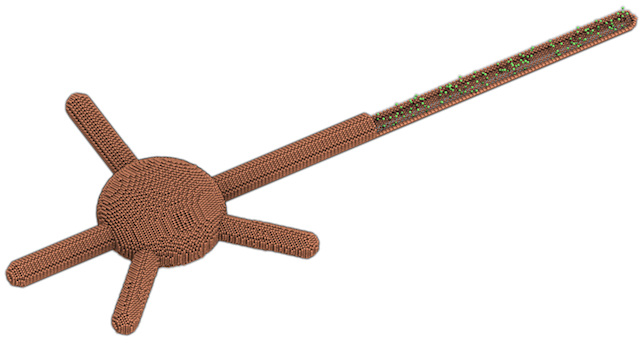Figure 7: A neuron-shaped compartment created from a combination of rod and ellipsoid compartment geometries. The model is created from the Python script shown in Figure 5.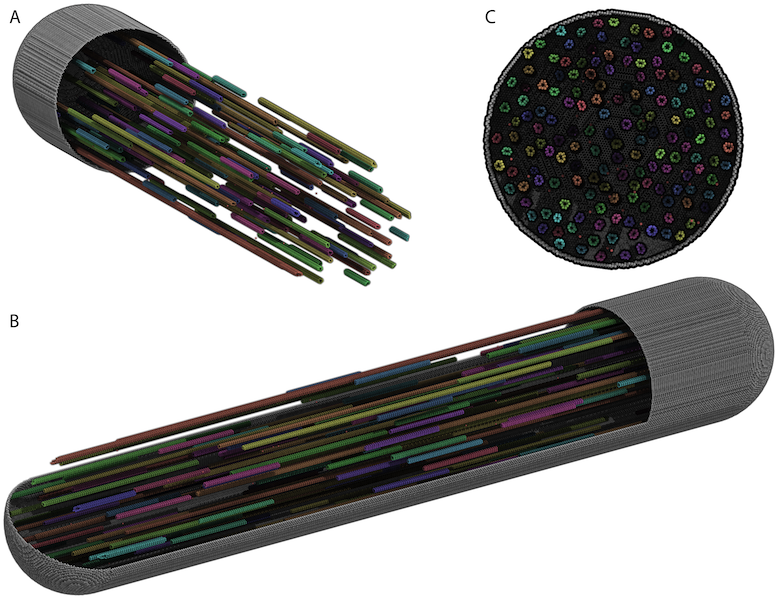Figure 8: A rod compartment containing randomly distributed microtubules built from cylinder compartments. The model is created from the Python script shown in Figure 6. The steps to create each of the displayed panels in SpatiocyteVisualizer are as follows: (A) (i) select all species (i.e., the default configuration), (ii) decrease the +x range to the desired level, (iii) deselect the membrane.VACANT species, (iv) increase the +x range to the maximum level, and (v) select the membrane.VACANT species; (B) the same steps as in (A) and increase -y range to the desired level; and (C) the same steps as in (A) and rotate to the desired angle.

### GEOMETRY¶

The GEOMETRY variable of a volume compartment specifies one of the six supported geometric primitives: cuboid (‘0’), ellipsoid (‘1’), cylinder (‘2’), rod (‘3’), pyramid (‘4’) and erythrocyte (‘5’). More complex forms can be constructed using a combination of these primitives. Figures 4 and 6 illustrate the construction of a neuron-shaped model using a combination of ellipsoid and rod compartments. Compartments without the GEOMETRY definition is set to the cuboid form since the default value is ‘0’. For examples of GEOMETRY definition see Figures 2 (line 4), 5 (lines 17 and 41) and 6 (lines 46 and 63).

### LENGTH[X, Y, Z]¶

The three variables LENGTH[X, Y, Z] can specify the compartment lengths in the directions of [x, y, z]-axes, respectively. The cuboid, ellipsoid and pyramid compartments use all three variables. If all three lengths are equal, a cube or a sphere compartment can be created with a cuboid or an ellipsoid geometry, respectively. For the pyramid compartment, LENGTH[X, Y, Z] stipulate its base length, height and base width, respectively. For a cylinder compartment, LENGTHX defines the cylinder length, while its diameter is given by LENGTHY. In the case of a rod compartment, LENGTHX indicates the length from the tip of one pole to the other while LENGTHY defines its diameter. For an erythrocyte, its width in the x and y directions are given by LENGTHX and LENGTHY respectively, whereas LENGTHZ determines its thickness. LENGTH[X, Y, Z] definitions examples are given in Figures 2 (lines 5-6), 5 (lines 5-7, 18-20, and 42-43) and 6 (lines 47-48 and 64-65).

### [XY, XZ, YZ]PLANE¶

When a volume compartment has the cuboid geometry, the boundary type or the presence of the [xy, xz, yz]-plane surfaces enclosing the compartment can be specified using [XY, XZ, YZ]PLANE variables. The boundary type can be reflective (‘0’), periodic (‘1’) or semi-periodic (‘2’). A semi-periodic boundary allows nonHD molecules to move unidirectionally from one boundary to the other. When a surface compartment is defined to enclose the cuboid compartment, we can remove one or both faces of the cuboid in a given [XY, XZ, YZ]PLANE. To remove the surface on the upper or the lower face of the cuboid in a plane, we can set the variable to ‘3’ or ‘4’, respectively, whereas to remove both faces we can set it to ‘5’. If the variable is not defined, the boundary type is set to the default reflective (‘0’) type. Examples in EM and Python to remove both of the cuboid XYPLANE faces are given below:

```Variable Variable(XYPLANE) { Value 5; }
theSimulator.createEntity('Variable', 'Variable:.:XYPLANE').Value = 5
```

### ORIGIN[X, Y, Z]¶

A child volume compartment can be placed at any location within a parent compartment using the variables ORIGIN[X, Y, Z]. The variables define the origin (center) coordinates of the child compartment relative to its parent center point. The variable values ‘-1’ and ‘1’ correspond to the normalized lowest and the highest points of the parent compartment in a given axis, respectively. Since the default value of these variables is ‘0’, the child compartment will be placed at the center of its parent if they are not defined. Figures 5 (lines 21-24 and 44-46) and 6 (lines 66-68) give some examples of the ORIGIN[X, Y, Z] variables definition.

### ROTATE[X, Y, Z]¶

A compartment can be rotated along the [x, y, z]-axis with the origin at the compartment center using the ROTATE[X, Y, Z] variables respectively. The unit of the variables is in radians. If there are multiple rotation definitions, they follow the [x, y, z]-axis rotation order. Compartments are not rotated if the variables are not defined since their default value is ‘0’. An example of compartment rotation definition is given in Figure 5 (line 47).

### VACANT¶

Every compartment must have a VACANT variable that represents the ‘species’ of empty voxels within the compartment. The VACANT voxels of a surface compartment are analogous to the lipid molecules mentioned in the Spatiocyte Method section and in (Arjunan and Tomita, 2010). Examples of the VACANT variable definition are shown in Figures 2 (lines 7 and 34), 5 (lines 8, 24, 32, 48 and 53) and 6 (lines 49, 60, 69 and 72). The variable can be used to define sink (e.g., A -> VACANT) and membrane binding reactions (e.g., BV + VACANTS -> BS) of nonHD species, as shown in the EM and Python examples below:

First-Order Sink Reaction, A →  Ø

```Process SpatiocyteNextReactionProcess(sink) {
VariableReferenceList [\_ Variable:/:A -1]
[\_ Variable:/:VACANT 1];
k 0.3; }
```

Second-Order Surface-Adsorption Reaction, Bv + Surface.VACANT → Bs

```Process DiffusionInfluencedReactionProcess(bind) {
VariableReferenceList [\_ Variable:/:B -1]
[\_ Variable:/Surface:VACANT -1]
[\_ Variable:/Surface:B 1];
k 2e-8; }
```

First-Order Sink Reaction, A →  Ø

```sinker = theSimulator.createEntity('SpatiocyteNextReactionProcess',
'Process:/:sink')
sinker.VariableReferenceList = [['\_', 'Variable:/:A', '-1']]
sinker.VariableReferenceList = [['\_', 'Variable:/:VACANT', '1']]
sinker.k = 0.3
```

Second-Order Surface-Adsorption Reaction, Bv + Surface.VACANT → Bs

```binder = theSimulator.createEntity('DiffusionInfluencedReactionProcess',
'Process:/:bind')
binder.VariableReferenceList = [['\_', 'Variable:/:B', '-1']]
binder.VariableReferenceList = [['\_', 'Variable:/Surface:VACANT',
'-1']]
binder.VariableReferenceList = [['\_', 'Variable:/Surface:B', '1']]
binder.k = 2e-8
```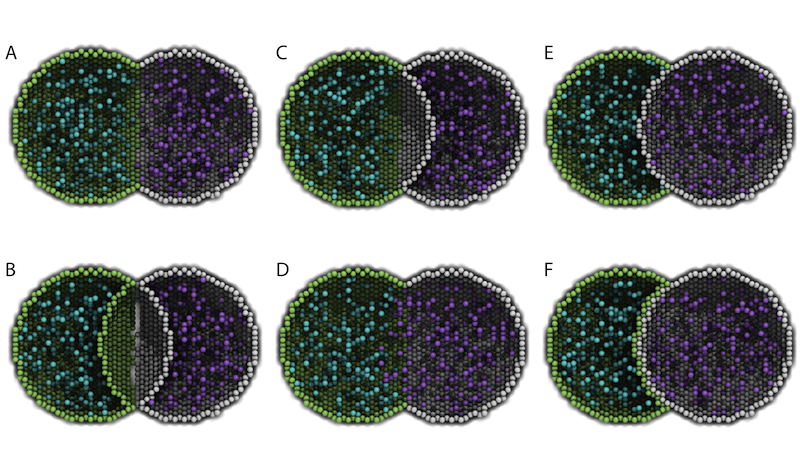Figure 9: Cross-sections of two intersected peer compartments. Two sphere compartments in green and white are intersecting in space. Turquoise and purple molecules belong to the green and white compartments respectively. See text of the VACANT variable and Table 1 for a detailed description of the intersections. The EM file to create the intersections is available in the Spatiocyte source package as 2012.arjunan.chapter.peer.em.

For a volume compartment, the Value of the VACANT variable determines if the compartment has a higher occupancy priority when it intersects with a peer compartment. Figure 9 displays cross-sections of various intersection forms of two spherical peer compartments with different volume and surface VACANT values (listed in Table 1). In the case of a surface compartment, the VACANT variable determines if it fully encloses a parent compartment that has an intersection. A nonzero value indicates that the parent will be fully enclosed even at the location of intersection. Otherwise if the value is ‘0’, the surface will be open at the intersecting region. Figure 10 shows four possible enclosure forms when a compartment intersects with a root compartment. Figure 7 illustrates the intersection of various compartments to create a unified neuron-shaped compartment.

Table 1: Combinations of volume and surface VACANT values and their corresponding intersected peer compartment forms. In all cases X is an integer and the DIFFUSIVE variable is not set.

 Green Sphere Compartment White Sphere Component Intersection Form in Figure 9 Volume VACANT.Value Surface VACANT.Value Volume VACANT.Value Surface VACANT.Value X 0 X 0 A X nonzero X nonzero B X 0 X nonzero C < X 0 X 0 D < X 0 X nonzero E

### DIFFUSIVE¶

To unify intersecting compartments, the DIFFUSIVE variable can be specified. It enables nonHD molecules to diffuse into and from an intersecting compartment. The Name property of the DIFFUSIVE variable defines the path and name of the diffusible intersecting compartment. With the DIFFUSIVE variable defined, the VACANT species of the unified compartments become identical. Figure 5 (lines 49 and 54) gives some examples of the DIFFUSIVE variable definition and usage.

### REACTIVE¶

The REACTIVE variable enables nonHD molecules in a surface compartment to collide and react with the VACANT voxels (i.e., lipids) and nonHD molecules in an adjacent surface compartment. The Name property of the REACTIVE variable specifies the path and name of the reactive adjacent surface compartment. Examples of the REACTIVE variable definition in EM and Python are given below:

```Variable Variable(REACTIVE) { Name "/Cell:Surface"; }
theSimulator.createEntity('Variable', 'Variable:/Surface:REACTIVE').Name
= "/Cell:Surface"
```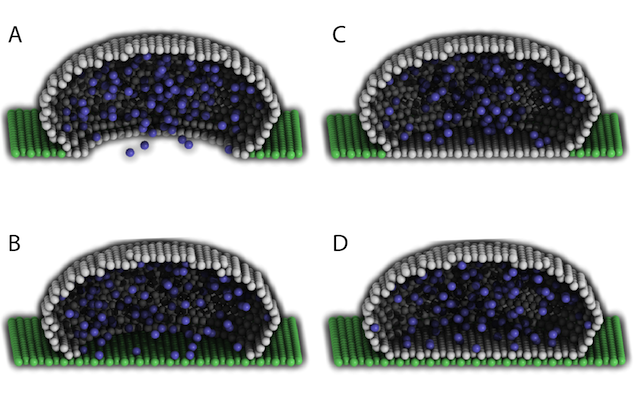Figure 10: Cross-sections of intersected root and child compartments. The VACANT surface voxels of the cuboid root compartment are shown in green while those of the ellipsoid child compartment are in white. The blue molecules belong to the child volume compartment. (A) root surface.VACANT = 0 and child surface.VACANT = 0, (B) root surface.VACANT = 1 and child surface.VACANT = 0, (C) root surface.VACANT = 0 and child surface.VACANT = 1, and (D) root surface.VACANT = 1 and child surface.VACANT = 1. The EM file to create the intersections is available in the Spatiocyte source package as 2012.arjunan.chapter.root.em.

## SpatiocyteStepper¶

The SpatiocyteStepper is the only stepper used by Spatiocyte in the E-Cell System and must be defined to run all simulations. It advances the simulation in an event-driven manner. Initialization examples of the SpatiocyteStepper are shown in Figures 2 (line 1), 5 (line 2) and 6 (line 44). In each compartment, the StepperID must be set to the SpatiocyteStepper ID. Examples of SpatiocyteStepper ID definition in compartments are given in Figures 2 (lines 3 and 32), 5 (lines 4, 16, 30, 40 and 51) and 6 (lines 45, 58, 62 and 70).

The radius of the HCP lattice voxels can be set in the SpatiocyteStepper using the VoxelRadius property. The default radius is 10e-9 m. Figures 2 (line 1), 5 (line 2) and 6 (line 44) show some examples of the VoxelRadius initialization.

### SearchVacant¶

The SearchVacant property of the SpatiocyteStepper provides an option to direct the simulator to search for all adjacent voxels for vacancy during dissociation reactions that result in nonHD product molecules. The reaction can only take place if there is an available target vacant voxel. This option is useful when evaluating the effects of a crowded compartment. The value of SearchVacant by default is false (‘0’). To enable it, we can set it to ‘1’. When disabled, an adjacent target voxel is selected randomly and the reaction is only executed if the voxel is vacant. EM and Python examples of SearchVacant initialization  are as follows:

Stepper SpatiocyteStepper(SS) { SearchVacant 0; }

theSimulator.createStepper(‘SpatiocyteStepper’, ‘SS’).SearchVacant = 0

## MoleculePopulateProcess¶

The initial positions of all nonHD species with nonzero initial molecule numbers must be specified with the MoleculePopulateProcess. The molecules can be either uniformly or normally distributed within the compartment. By default, without any MoleculePopulateProcess parameter definition, molecules are uniformly distributed over the entire compartment. Otherwise if the GaussianSigma is set to a nonzero value,  the compartment will be populated according to the Gaussian distribution. MoleculePopulateProcess definitions can be seen in Figures 2 (lines 26-28), 5 (lines 13-14) and 6 (line 57). A Python example showing two different species populated at the poles of a rod surface compartment is also listed in Figure 11 with the corresponding output in Figure 12.

 ``` 1 2 3 4 5 6 7 8 9 10 11 12 13 14 15 16 17 18 19 20 21 22 23 24 25 26``` ```# Example of python scripting to populate molecules at the poles of a rod compartment theSimulator.createStepper('SpatiocyteStepper', 'SS').VoxelRadius = 8e-8 # Create the root container compartment using the rod geometry: theSimulator.rootSystem.StepperID = 'SS' theSimulator.createEntity('Variable', 'Variable:/:GEOMETRY').Value = 3 theSimulator.createEntity('Variable', 'Variable:/:LENGTHX').Value = 10e-6 theSimulator.createEntity('Variable', 'Variable:/:LENGTHY').Value = 2e-6 theSimulator.createEntity('Variable', 'Variable:/:VACANT') logger = theSimulator.createEntity('VisualizationLogProcess', 'Process:/:logger') logger.LogInterval = 1 logger.VariableReferenceList = [['\_', 'Variable:/Surface:A'], ['\_', 'Variable:/Surface:B']] populator = theSimulator.createEntity('MoleculePopulateProcess', 'Process:/:populateLeft') populator.VariableReferenceList = [['\_', 'Variable:/Surface:A']] populator.OriginX = -1 populator.UniformLengthX = 0.5 populator = theSimulator.createEntity('MoleculePopulateProcess', 'Process:/:populateRight') populator.VariableReferenceList = [['\_', 'Variable:/Surface:B']] populator.OriginX = 1 populator.UniformLengthX = 0.5 # Create the surface compartment: theSimulator.createEntity('System', 'System:/:Surface').StepperID = 'SS' theSimulator.createEntity('Variable', 'Variable:/Surface:DIMENSION').Value = 2 theSimulator.createEntity('Variable', 'Variable:/Surface:VACANT') theSimulator.createEntity('Variable', 'Variable:/Surface:A').Value = 500 theSimulator.createEntity('Variable', 'Variable:/Surface:B').Value = 500 run(100) ```

Figure 11: A Python script to populate molecules at the poles of a rod surface compartment. The file is available in the Spatiocyte source package as 2012.arjunan.chapter.populate.py.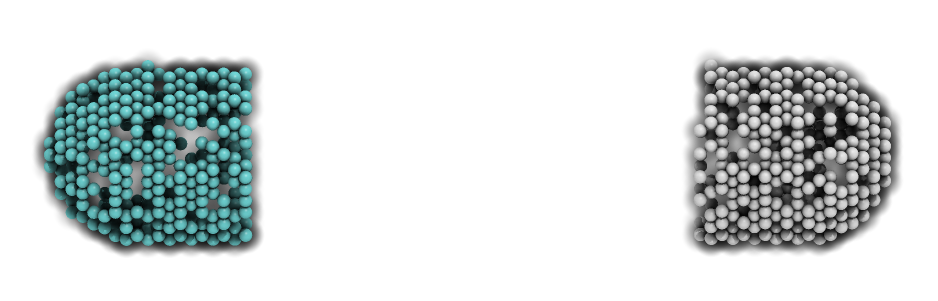Figure 12: Visualization of molecules populated at the poles of a rod surface compartment. The model is created from the Python script shown in Figure 11.

### Priority¶

Priority determines the order to populate multiple species using multiple MoleculePopulateProcess. This is necessary when the population of a species takes precedence over other species. The value of Priority is an integer which determines the priority of the process in the sequence. A higher value of Priority denotes a higher priority in the sequence. The default value of Priority is 0.

### Origin[X, Y, Z]¶

Origin[X, Y, Z] is the origin point relative to the compartment center point for a species population. The molecules may have a uniform or a Gaussian distribution from this point. The range of the point along each axis covering the entire compartment is [-1, 1]. Therefore, the origin is at the center of the compartment if Origin[X, Y, Z] is fixed to [0, 0, 0], the default set of values.

### GaussianSigma[X, Y, Z]¶

GaussianSigma[X, Y, Z] stipulates the sigma value for a Gaussian distributed population from the origin in [x, y, z]-axis, respectively.

### UniformLength[X, Y, Z]¶

The uniformly distributed normalized population radius from the origin point in [x, y, z]-axis is given by the UniformLength[X, Y, Z] parameter. Since the default values of UniformLength[X, Y, Z] and Origin[X, Y, Z] are [1, 1, 1] and [0, 0, 0], respectively, the molecules are spread uniformly within the entire compartment when the parameters are not defined.

### ResetTime¶

To place the molecules at a certain interval after the simulation has started, we can use the ResetTime parameter. This parameter is useful when the positions of a molecule species need to be actively altered after a simulation interval.

## DiffusionProcess¶

The DiffusionProcess handles the voxel-to-voxel random walk of diffusing molecules and the collisions that take place between each walk. A DiffusionProcess can diffuse multiple species having the same diffusion coefficient and within the same compartment. The VariableReference coefficient of the diffusing species must be set to 0, the default value. We can set a species to diffuse only over a designated species (i.e., it acts as a vacant species to the diffusing species) by including the designated species in the VariableReference list and setting its coefficient to -1. Examples of the DiffusionProcess usage are shown in Figures 2 (lines 11-16 and 39-50), 5 (lines 26-28) and 6 (lines 51-53). Below is a Python example to diffuse A molecules over B molecules with a diffusion coefficient of 1e-12  m2s-1.

```diffuser = theSimulator.createEntity('DiffusionProcess',
'Process:/:diffuse')
binder.VariableReferenceList = [['\_', 'Variable:/Surface:A']]
binder.VariableReferenceList = [['\_', 'Variable:/Surface:B', '-1']]
binder.D = 1e-12
```

### D¶

In the DiffusionProcess, the diffusion coefficient of the molecule species is set with D, which has the unit m2s-1. The default value is 0 m2s-1.

### P¶

P is an arbitrarily set reaction probability limit of the diffusing species, within the range [0, 1]. The default value is ‘1’, which is sufficient to produce accurate simulations. We can set it to a smaller value  to perform reaction-diffusion processes at smaller intervals.

## PeriodicBoundaryDiffusionProcess¶

We can use the PeriodicBoundaryDiffusionProcess in place of the DiffusionProcess when a molecule species needs to be diffused across periodic two-dimensional surface edges. The surface compartment must be enclosing a cuboid parent compartment. The process overcomes the limitation of setting  [XY, XZ, YZ]PLANE of the Compartment variable to periodic, which only supports periodic volume edges. It inherits the diffusion coefficient, D and the reaction probability limit, P from the DiffusionProcess. Examples of PeriodicBoundaryDiffusionProcess in EM and Python are as follows:

```Process PeriodicBoundaryDiffusionProcess(diffuse) {
VariableReferenceList [\_ Variable:/Surface:A];
D 0.2e-12; }

diffuser = theSimulator.createEntity('PeriodicBoundaryDiffusionProcess',
'Process:/:diffuse')
diffuser.VariableReferenceList = [['\_', 'Variable:/Surface:A']]
diffuser.D = 0.2e-12
```

## DiffusionInfluencedReactionProcess¶

The DiffusionInfluencedReactionProcess is used to execute all second-order reactions comprising two diffusing reactants, or a diffusing and an immobile reactant (Reactant 1 and Reactant 2 are nonHD molecules). Figure 2 (lines 51-60 and lines 67-69) shows several usage examples of DiffusionInfluencedReactionProcess. A python example of the process definition is provided below:

Second-Order Reaction, A + B → C

```binder = theSimulator.createEntity('DiffusionInfluencedReactionProcess',
'Process:/:associate')
binder.VariableReferenceList = [['\_', 'Variable:/:A', '-1']]
binder.VariableReferenceList = [['\_', 'Variable:/:B', '-1']]
binder.VariableReferenceList = [['\_', 'Variable:/:C', '1']]
binder.p = 0.5
```

### k¶

The intrinsic rate constant of the diffusion-influenced reaction is set to k. In volume reactions, the relationship between the intrinsic rate constant with the macroscopic rate constant kon is given by 1/kon = 1/k + 1/kd, where kd= 4πDR is the maximally diffusion-limited reaction rate, D is the diffusion coefficient and R is the contact radius (i.e., 2rv). The units of k for various reaction types are given in Table 2.

### p¶

The absolute reactive collision probability of the reaction is given by p. This process requires either the value of k or p.

Table 2: Units of the rate constant, k in *DiffusionInfluencedReactionProcess (Reactant 1 and Reactant 2 are nonHD) and †SpatiocyteNextReactionProcess (Reactant 1 and/or Reactant 2 are HD).

Reactant1 Reactant2 Product1 Product2 k(units)
*†Volume Volume Volume Volume m3s-1
*†Surface Surface Surface Surface m2s-1
*†Volume Surface Volume Volume m2s-1
*†Volume Surface Surface Surface m3s-1
*†Volume Surface Volume Surface m3s-1
*†Volume Surface,VACANT Surface None ms-1
†Volume None Surface None ms-1
†Volume None Volume None s-1
†Surface None Surface None s-1
†Surface None Volume None s-1

## SpatiocyteNextReactionProcess¶

The SpatiocyteNextReactionProcess is used to execute all reactions that can be decoupled from diffusion such as zeroth- and first-order reactions, and second-order reactions that involve two adjoining immobile reactants or at least one HD reactant. Each reaction is performed according to the Next Reaction method (Gibson and Bruck, 2000). Unlike in the DiffusionInfluencedReactionProcess, the membrane-adsorption reaction where a HD species binds to the membrane is represented as a first-order reaction (see example below). EM examples of the SpatiocyteNextReactionProcess are given in Figure 2 (lines 61-66 and 70-75), while Python examples of zeroth- and first-order (surface-adsorption) reactions are given below:

Zeroth-Order Reaction, 1 → A

```zero = theSimulator.createEntity('SpatiocyteNextReactionProcess',
'Process:/:create')
zero.VariableReferenceList = [['\_', 'Variable:/:A', '1']]
zero.k = 0.01
```

First-Order Surface-Adsorption Reaction, Av → As

```uni = theSimulator.createEntity('SpatiocyteNextReactionProcess',
uni.VariableReferenceList = [['\_', 'Variable:/:A', '-1']]
uni.VariableReferenceList = [['\_', 'Variable:/Surface:A', '1']]
uni.k = 0.01
```

### k¶

The rate constant of the event-driven reaction. For second-order reactions, the units are listed in Table 2. In the case of the intercompartmental surface-adsorption reaction, the unit is in ms-1. For all other first-order reactions the unit is in s-1.

### Space[A, B, C]¶

Sometimes the size of the compartment containing the reacting species is too large and all the molecules within the compartment are HD species. To avoid unnecessarily allocating a large amount of memory to represent the compartment that are unpopulated with any nonHD species, we can override the declared size of the compartment with the variables Space[A, B, C]. SpaceA and SpaceB correspond to the size of the compartment containing the first and second reactants respectively, whereas SpaceC denotes the size of the product compartment. The units of Space[A, B, C] correspond to the dimensions of the respective compartment. By default, the values of Space[A, B, C] are set to zero. Only a nonzero positive value will override the respective compartment size.

## VisualizationLogProcess¶

We can use the VisualizationLogProcess to log the coordinates of nonHD species at a specified periodic interval. The SpatiocyteVisualizer can load the log file to display the molecules in 3D. Figures 2 (lines 17-20), 5 (lines 9-12) and 6 (lines 54-56 and 73) show some examples of VisualizationLogProcess usage.

### FileName¶

FileName is the name of the binary log file. The default name is ‘visualLog0.dat’, which is also the default file name loaded by SpatiocyteVisualizer.

The interval for logging the coordinates is determined by LogInterval. The default value is ‘0’, which means that the interval would be set to the smallest diffusion or collision interval of the logged nonHD species. If LogInterval > 0,  the log interval will be set to the specified value. The unit of LogInterval is in seconds.

## MicroscopyTrackingProcess¶

The MicroscopyTrackingProcess mimics the fluorescent microphotography process by logging the trajectory of nonHD molecules averaged over a specified camera exposure time. It inherits the FileName and LogInterval properties from the VisualizationLogProcess. After each LogInterval, the number of times a voxel is occupied by a molecule species is counted. At the end of a given ExposureTime, the frequency is averaged over the total number of intervals and logged. Figure 2 (lines 21-25) shows an example of the MicroscopyTrackingProcess definition. A Python example is given below:

```tracker = theSimulator.createEntity('MicroscopyTrackingProcess',
'Process:/:track')
tracker.VariableReferenceList = [['\_', 'Variable:/Surface:MinEE', '2']]
tracker.VariableReferenceList = [['\_', 'Variable:/Surface:MinDEE',
'3']]
tracker.VariableReferenceList = [['\_', 'Variable:/Surface:MinE', '-2']]
tracker.VariableReferenceList = [['\_', 'Variable:/Surface:MinDE',
'-2']]
tracker.VariableReferenceList = [['\_', 'Variable:/Surface:MinE', '-1']]
tracker.FileName = “microscopyLog0.dat”
```

MicroscopyTrackingProcess enables representation of different fluorescent colored subunits within a complex according to the coefficient assigned to each variable. In the Python example above, the coefficient of the first variable MinEE is 2, representing two subunits of MinE within the complex MinEE. Similarly for MinDEE, the three subunits (one MinD and two MinE’s) are represented by the coefficient 3. Each unique variable with a negative coefficient is assigned a different color during visualization. The first negative variable, MinE, has a coefficient of -2, which means that two subunits from the first positive variable, MinEE, are assigned a unique color of MinE. The second negative  variable MinDE also has a coefficient of -2, specifying that two subunits of the second positive variable, MinDEE, is assigned the color of MinDE. The third negative variable MinE has a coefficient of -1, corresponding to the color of the remaining one MinE subunit of the second positive variable MinDEE.

### ExposureTime¶

The simulated camera exposure time is specified by ExposureTime. The default value is 0.5 s.

### MeanCount¶

MeanCount is the maximum number of voxel occupancy frequency before it is averaged. The default value is ‘0’, which indicates that the specified LogInterval or the smallest collision or diffusion interval should be used. In this case, the MeanCount will be ExposureTime/LogInterval. Otherwise if MeanCount > 0, the LogInterval is set to ExposureTime/MeanCount.

## IteratingLogProcess¶

The IteratingLogProcess executes multiple simulation runs with different random seeds and logs the averaged physical values of molecules, such as their displacement or survival probability, over the total runs. The values are logged in a file using the comma-separated values (csv) format. By default the process logs the number of available molecules of recorded species at the specified interval periodically.

### LogDuration¶

LogDuration is the total duration of a simulation run (i.e., an iteration).

LogInterval is the interval for logging physical values of molecules within an iteration.

### Iterations¶

The number of simulation runs before the logged values are averaged and saved in the log file is specified by the Iterations parameter.

### FileName¶

The file name of the log file is given by FileName. The default file name is “Log.csv”.

### SaveInterval¶

When running many iterations, it is useful to save the logged data in a backup file for quick analysis, or to avoid restarting the runs because of some unexpected failures (e.g., power failure). To this end, a backup file of the logged values can be saved at the iteration intervals given by Iterations/SaveInterval. The default value of SaveInterval is ‘0’, which indicates that a backup file will not be saved.

### Survival¶

The Survival parameter can be set to ‘1’ to log the survival probability of a molecule species. The default value of the parameter is ‘0’.

### Displacement¶

Set the Displacement  to ‘1’ to log the displacement of a molecule species.  The default value of Displacement is ‘0’.

### Diffusion¶

If the Diffusion parameter is set to ‘1’, the apparent diffusion coefficient of a molecule species will be logged. The default Diffusion value is ‘0’.

## SpatiocyteVisualizer¶

The SpatiocyteVisualizer can be started by executing spatiocyte in any directory. Figure 3 illustrates the SpatiocyteVisualizer interface, while its features and keyboard shortcuts are listed in Table 3. To change the color of a species, right mouse click on the species and select a desired color. The visualizer can display each species within a specified range in each axis using the bounding feature. Figure 8 displays the output after specifying a set of ranges for the cell membrane. Each displayed frame can be saved into the Portable Network Graphics (PNG) image format. A quick way to create a movie from the saved images is to use the ffmpeg program:

```\$ avconv -i image%07d.png -vcodec qtrle out.mov
```

Table 3: SpatiocyteVisualizer features and keyboard shortcuts

Feature Keyboard shortcut(s)
Play Forward Right arrow
Play Backward Left arrow
Step Forward Up arrow or Enter
Step Backward Down arrow or Shift+Enter
Pause/Play Space
Zoom In f or Ctrl++ or Ctrl+= or PageUp
Zoom Out b or Ctrl+- or PageDown
Reset View Ctrl+0 or Home
Rotate along x-axis clockwise Ctrl+Up Arrow
Rotate along x-axis counter-clockwise Ctrl+Down Arrow
Rotate along y-axis clockwise Ctrl+Right Arrow
Rotate along y-axis counter-clockwise Ctrl+Left Arrow
Rotate along z-axis clockwise z
Rotate along z-axis counter-clockwise Z
Translate Up Shift+Up Arrow
Translate Down Shift+Down Arrow
Translate Right Shift+Right Arrow
Translate Left Shift+Left Arrow
Translate Front F
Translate Back B
Save current frame as a PNG image s
Start/Stop recording PNG frames S